# Evaluation Metrics for Language Modeling

Recently, neural network trained language models, such as ULMFIT, BERT, and GPT-2, have been remarkably successful when transferred to other natural language processing tasks. As such, there's been growing interest in language models.

Traditionally, language model performance is measured by perplexity, cross entropy, and bits-per-character (BPC). As language models are increasingly being used as pre-trained models for other NLP tasks, they are often also evaluated based on how well they perform on downstream tasks. The GLUE benchmark score is one example of broader, multi-task evaluation for language models .

Counterintuitively, having more metrics actually makes it harder to compare language models, especially as indicators of how well a language model will perform on a specific downstream task are often unreliable. One of my favorite interview questions is to ask candidates to explain perplexity or the difference between cross entropy and BPC. While almost everyone is familiar with these metrics, there is no consensus: the candidates’ answers differ wildly from each other, if they answer at all.

One point of confusion is that language models generally aim to minimize perplexity, but what is the lower bound on perplexity that we can get since we are unable to get a perplexity of zero? If we don’t know the optimal value, how do we know how good our language model is?

Moreover, unlike metrics such as accuracy where it is a certainty that 90% accuracy is superior to 60% accuracy on the same test set regardless of how the two models were trained, arguing that a model’s perplexity is smaller than that of another does not signify a great deal unless we know how the text is pre-processed, the vocabulary size, the context length, etc. For instance, while perplexity for a language model at character-level can be much smaller than perplexity of another model at word-level, it does not mean the character-level language model is better than that of the word-level. Therefore, how do we compare the performance of different language models that use different sets of symbols? Or should we?

Despite the presence of these downstream evaluation benchmarks, traditional intrinsic metrics are, nevertheless, extremely useful during the process of training the language model itself. In this article, we will focus on those intrinsic metrics. We will accomplish this by going over what those metrics mean, exploring the relationships among them, establishing mathematical and empirical bounds for those metrics, and suggesting best practices with regards to how to report them.

# Understanding perplexity, bits-per-character, and cross entropy

## Language model

Consider an arbitrary language $L$. In this case, English will be utilized to simplify the arbitrary language. A language model assigns probabilities to sequences of arbitrary symbols such that the more likely a sequence $(w_1, w_2, ..., w_n)$ is to exist in that language, the higher the probability. A symbol can be a character, a word, or a sub-word (e.g. the word ‘going’ can be divided into two sub-words: ‘go’ and ‘ing’). Most language models estimate this probability as a product of each symbol's probability given its preceding symbols:Probability of a sentence can be defined as the product of the probability of each symbol given the previous symbols Alternatively, some language models estimate the probability of each symbol given its neighboring symbols, also known as the cloze task. In this article, we refer to language models that use Equation (1).

## Entropy

The goal of any language is to convey information. To measure the average amount of information conveyed in a message, we use a metric called “entropy", proposed by Claude Shannon . It should be noted that entropy in the context of language is related to, but not the same as, entropy in the context of thermodynamics.

An intuitive explanation of entropy for languages comes from Shannon himself in his landmark paper “Prediction and Entropy of Printed English" :

The entropy is a statistical parameter which measures, in a certain sense, how much information is produced on the average for each letter of a text in the language. If the language is translated into binary digits (0 or 1) in the most efficient way, the entropy is the average number of binary digits required per letter of the original language."

We know that for 8-bit ASCII, each character is composed of 8 bits. However, this is not the most efficient way to represent letters in English language since all letters are represented using the same number of bits regardless of how common they are (a more optimal scheme would be to use less bits for more common letters). Thus, we should expect that the character-level entropy of English language to be less than 8.

Shannon approximates any language’s entropy $H$ through a function $F_N$ which measures the amount of information, or in other words, entropy, extending over $N$ adjacent letters of text. Let $b_n$ represents a block of $n$ contiguous letters $(w_1, w_2, ..., w_n)$.Define the function $K_N = -\sum\limits_{b_n}p(b_n)\textrm{log}_2p(b_n)$, we have:Shannon defined language entropy $H$ to be:Note that by this definition, entropy is computed using an infinite amount of symbols. In practice, we can only approximate the empirical entropy from a finite sample of text.

## Cross entropy

Owing to the fact that there lacks an infinite amount of text in the language $L$, the true distribution of the language is unknown. A language model aims to learn, from the sample text, a distribution $Q$ close to the empirical distribution $P$ of the language. In order to measure the “closeness" of two distributions, cross entropy is often used. Mathematically. the cross entropy of Q with respect to P is defined as follows: $$\textrm{H(P, Q)} = \textrm{E}_{P}[-\textrm{log} Q]$$

When P and Q are discrete, this becomes: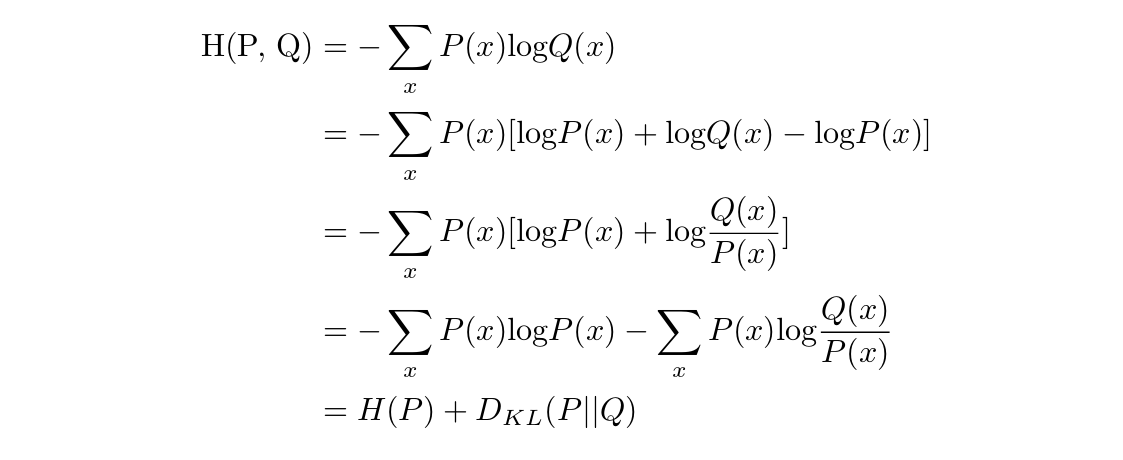with $D_{KL}(P || Q)$ being the Kullback–Leibler (KL) divergence of Q from P. This term is also known as relative entropy of P with respect to Q. Therefore, the cross entropy of Q with respect to P is the sum of the following two values:

1. the average number of bits needed to encode any possible outcome of P using the code optimized for P [which is $H(P)$ - entropy of P].

2. the number of extra bits required to encode any possible outcome of P using the code optimized for Q.

It should be noted that since the empirical entropy $H(P)$ is unoptimizable, when we train a language model with the objective of minimizing the cross entropy loss, the true objective is to minimize the KL divergence of the distribution, which was learned by our language model from the empirical distribution of the language.

## Perplexity

For a long time, I dismissed perplexity as a concept too perplexing to understand -- sorry, can’t help the pun. Wikipedia defines perplexity as:

a measurement of how well a probability distribution or probability model predicts a sample."

Intuitively, perplexity can be understood as a measure of uncertainty. The perplexity of a language model can be seen as the level of perplexity when predicting the following symbol. Consider a language model with an entropy of three bits, in which each bit encodes two possible outcomes of equal probability. This means that when predicting the next symbol, that language model has to choose among $2^3 = 8$ possible options. Thus, we can argue that this language model has a perplexity of 8.

Mathematically, the perplexity of a language model is defined as:

$$\textrm{PPL}(P, Q) = 2^{\textrm{H}(P, Q)}$$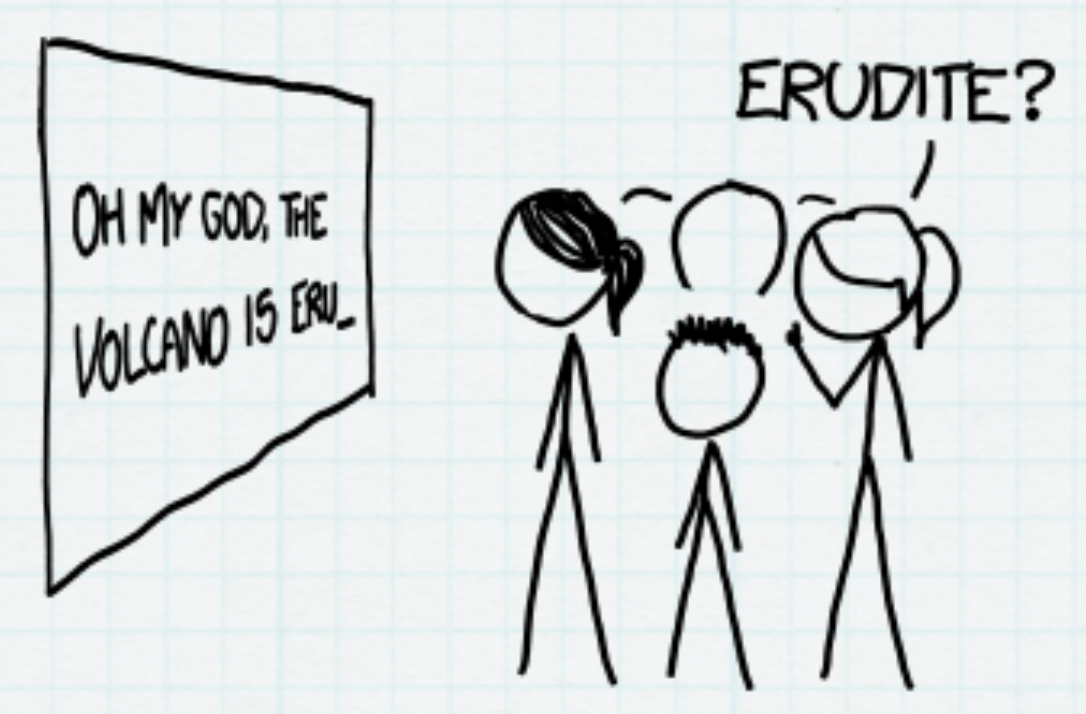If a human was a language model with statistically low cross entropy. Source: xkcd

## Bits-per-character and bits-per-word

Bits-per-character (BPC) is another metric often reported for recent language models. It measures exactly the quantity that it is named after: the average number of bits needed to encode on character. This leads to revisiting Shannon’s explanation of entropy of a language:

if the language is translated into binary digits (0 or 1) in the most efficient way, the entropy is the average number of binary digits required per letter of the original language."

By this definition, entropy is the average number of BPC. The reason that some language models report both cross entropy loss and BPC is purely technical.

While entropy and cross entropy are defined using log base 2 (with "bit" as the unit), popular machine learning frameworks, including TensorFlow and PyTorch, implement cross entropy loss using natural log (the unit is then nat). This is due to the fact that it is faster to compute natural log as opposed to log base 2. In theory, the log base does not matter because the difference is a fixed scale: $$\frac{\textrm{log}_e n}{\textrm{log}_2 n} = \frac{\textrm{log}_e 2}{\textrm{log}_e e} = \textrm{ln} 2$$

Suggestion: In practice, if everyone uses a different base, it is hard to compare results across models. For the sake of consistency, I urge that, when we report entropy or cross entropy, we report the values in bits.

Keep in mind that BPC is specific to character-level language models. When we have word-level language models, the quantity is called bits-per-word (BPW) – the average number of bits required to encode a word. The relationship between BPC and BPW will be discussed further in the section [across-lm].

# Reasoning about entropy as a metric

Since we can convert from perplexity to cross entropy and vice versa, from this section forward, we will examine only cross entropy.

For many of metrics used for machine learning models, we generally know their bounds. For example, the best possible value for accuracy is 100% while that number is 0 for word-error-rate and mean squared error. If our model reaches 99.9999% accuracy, we know, with some certainty, that our model is very close to doing as well as it is possibly able.

When it is argued that a language model has a cross entropy loss of 7, we do not know how far it is from the best possible result if we do not know what the best possible result should be. Not knowing what we are aiming for can make it challenging in regards to deciding the amount resources to invest in hopes of improving the model.

## Mathematical bounds

It is imperative to reflect on what we know mathematically about entropy and cross entropy. Firstly, we know that the smallest possible entropy for any distribution is zero. However, the entropy of a language can only be zero if that language has exactly one symbol.If a language has two characters that appear with equal probability, a binary system for instance, its entropy would be: $$\textrm{H(P)} = - 0.5 * \textrm{log}(0.5) - 0.5 * \textrm{log}(0.5) = 1$$

Secondly, we know that the entropy of a probability distribution is maximized when it is uniform. This alludes to the fact that for all the languages that share the same set of symbols (vocabulary), the language that has the maximal entropy is the one in which all the symbols appear with equal probability.

Let $|\textrm{V}|$ be the vocabulary size of an arbitrary language with the distribution P.If we consider English as a language with 27 symbols (the English alphabet plus space), its character-level entropy will be at most: $$\textrm{log}(27) = 4.7549$$ According to , an average 20-year-old American knows 42,000 words, so their word-level entropy will be at most: $$\textrm{log}(42,000) = 15.3581$$

Thirdly, we understand that the cross entropy loss of a language model will be at least the empirical entropy of the text that the language model is trained on. If the underlying language has the empirical entropy of 7, the cross entropy loss will be at least 7.

Proof: let P be the distribution of the underlying language and Q be the distribution learned by a language model. By definition:Since ${D_{KL}(P || Q)} \geq 0$, we have:Lastly, remember that, according to Shannon’s definition, entropy is $F_N$ as $N$ approaches infinity. We will show that as $N$ increases, the $F_N$ value decreases. Intuitively, this makes sense since the longer the previous sequence, the less confused the model would be when predicting the next symbol.

We will confirm this by proving that $F_{N+1} \leq F_{N}$ for all $N \geq 1$. Assume that each character $w_i$ comes from a vocabulary of m letters ${x_1, x_2, ..., x_m}$. Remember that $F_N$ measures the amount of information or entropy due to statistics extending over N adjacent letters of text.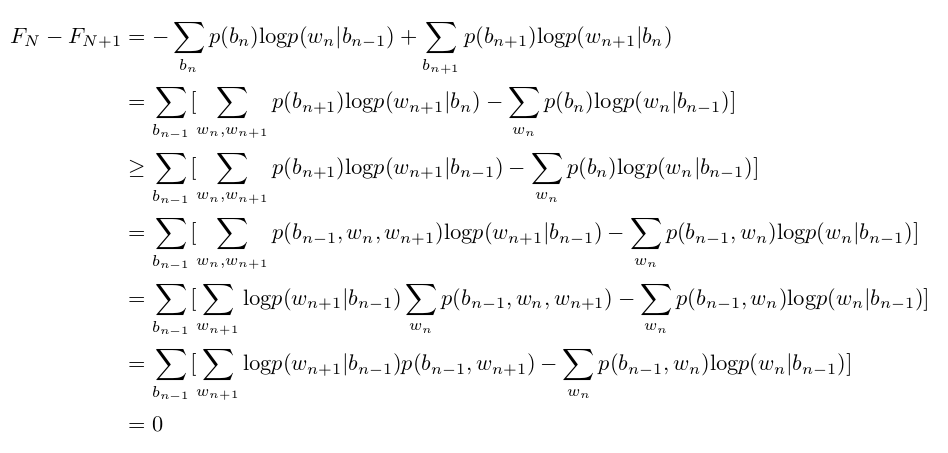The equality on the third line is because $\textrm{log}p(w_{n+1} | b_{n}) \geq \textrm{log}p(w_{n+1} | b_{n-1})$. The last equality is because $w_n$ and $w_{n+1}$ come from the same domain.

Therefore:This means that with an infinite amount of text, language models that use longer context length in general should have lower cross entropy value compared to those with shorter context length. An example of this can be a language model that uses a context length of 32 should have a lower cross entropy than a language model that uses a context length of 24. For a finite amount of text, this might be complicated because the language model might not see longer sequence enough to make meaningful predictions.

Suggestion: When reporting perplexity or entropy for a LM, we should specify the context length.

## Estimated bounds

Since the year 1948, when the notion of information entropy was introduced, estimating the entropy of the written English language has been a popular musing subject for generations of linguists, information theorists, and computer scientists. There are two main methods for estimating entropy of the written English language: human prediction and compression.

### Human predictions

This method assumes that speakers of any language possesses an enormous amount of statistical knowledge of that language, enabling them to guess the next symbol based on the preceding text.

Based on the number of guesses until the correct result, Shannon derived the upper and lower bound entropy estimates. He chose 100 random samples, each containing 100 characters, from Dumas Malone’s Jefferson the Virginian, the first volume in a Pulitzer prize-winning series of six titled Jefferson and His Time. He used both the alphabet of 26 symbols (English alphabet) and 27 symbols (English alphabet + space) [3:1]. See Table 1: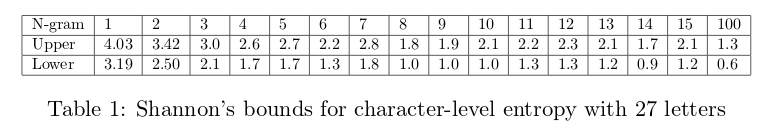Cover and King framed prediction as a gambling problem. They let the subject “wager a percentage of his current capital in proportion to the conditional probability of the next symbol." If the subject divides his capital on each bet according to the true probability distribution of the next symbol, then the true entropy of the English language can be inferred from the capital of the subject after $n$ wagers. They used 75-letter sequences from Dumas Malone’s Jefferson the Virginian and 220-letter sequences from Leonard and Natalie Zunin’s Contact: The First Four Minutes with a 27-letter alphabet . See Table 2:### Compression

Outside the context of language modeling, BPC establishes the lower bound on compression. If a text has BPC of 1.2, it can not be compressed to less than 1.2 bits per character. For example, if the text has 1000 characters (approximately 1000 bytes if each character is represented using 1 byte), its compressed version would require at least 1200 bits or 150 bytes.

Conversely, if we had an optimal compression algorithm, we could calculate the entropy of the written English language by compressing all the available English text and measure the number of bits of the compressed data.

In 1996, Teahan and Cleary used prediction by partial matching (PPM), an adaptive statistical data compression technique that uses varying lengths of previous symbols in the uncompressed stream to predict the next symbol . Utilizing fixed models of order five (using up to five previous symbols for prediction) and a 27-symbol alphabet, Teahan and Cleary were able to achieve BPC of 1.461 on the last chapter of Dumas Malone’s Jefferson the Virginian. 

In 2006, the Hutter prize was launched with the goal of compressing enwik8, the first 100MB of a specific version of English Wikipedia . As of April 2019, the winning entry continues to be held by Alexander Rhatushnyak with the compression factor of 6.54, which translates to about 1.223 BPC. In January 2019, using a neural network architecture called Transformer-XL, Dai et al. trained a language model to achieve BPC of 0.99 on enwik8 .

Table 3 shows the estimations of the entropy using two different methods:

## Comparing perplexities across language models

Until this point, we have explored entropy only at the character-level. However, there are also word-level and subword-level language models, which leads us to ponder surrounding questions. Is it possible to compare the entropies of language models with different symbol types? In other words, can we convert from character-level entropy to word-level entropy and vice versa?

Graves used this simple formula: if on average, a word requires $m$ bits to encode and a word contains $l$ characters, it should take on average $\frac{m}{l}$ bits to encode a character. In his paper Generating Sequences with Recurrent Neural Networks, because a word on average has 5.6 characters in the dataset, the word-level perplexity is calculated using: $2^{5.6 * \textrm{BPC}}$. 

Shannon used similar reasoning. Through Zipf’s law, which states that “the frequency of any word is inversely proportional to its rank in the frequency table", Shannon approximated the frequency of words in English and estimated word-level $F_1$ to be 11.82. Estimating that the average English word length to be 4.5, one might be tempted to apply the value $\frac{11.82}{4.5} = 2.62$ to be between the character-level $F_{4}$ and $F_{5}$.

However, $2.62$ is actually between character-level $F_{5}$ and $F_{6}$. The reason, Shannon argued, is that “a word is a cohesive group of letters with strong internal statistical influences, and consequently the N-grams within words are restricted than those which bridge words." [3:2]

For the value of $F_N$ for word-level with $N \geq 2$, the word boundary problem no longer exists as space is now part of the multi-word phrases. Therefore, if our word-level language models deal with sequences of length $\geq$ 2, we should be comfortable converting from word-level entropy to character-level entropy through dividing that value by the average word length.

The calculations become more complicated once we have subword-level language models as the space boundary problem resurfaces. We can convert from subword-level entropy to character-level entropy using the average number of characters per subword if you’re mindful of the space boundary.

Suggestion: When reporting perplexity or entropy for a LM, we should specify whether it is word-, character-, or subword-level.

# Empirical entropy

In this section, we will calculate the empirical character-level and word-level entropy on the datasets SimpleBooks, WikiText, and Google Books.

WikiText is extracted from the list of knowledgeable and featured articles on Wikipedia. It contains 103 million word-level tokens, with a vocabulary of 229K tokens. The vocabulary contains only tokens that appear at least 3 times – rare tokens are replaced with the $<$unk$>$ token. 

Created from 1,573 Gutenberg books with high length-to-vocabulary ratio, SimpleBooks has 92 million word-level tokens but with the vocabulary of only 98K and $<$unk$>$ token accounting for only 0.1%.

The Google Books dataset is from over 5 million books published up to 2008 that Google has digitialized. It is available as word N-grams for $1 \leq N \leq 5$. We examined all of the word 5-grams to obtain character N-gram for $1 \leq N \leq 9$.

We removed all N-grams that contain characters outside the standard 27-letter alphabet from these datasets. For the Google Books dataset, we analyzed the word-level 5-grams to obtain character N-gram for $1 \leq N \leq 9$. See Table 4, Table 5, and Figure 3 for the empirical entropies of these datasets.

The first thing to note is how remarkable Shannon’s estimations of entropy were, given the limited resources he had in 1950. Most of the empirical F-values fall precisely within the range that Shannon predicted, except for the 1-gram and 7-gram character entropy. Shannon’s estimation for 7-gram character entropy is peculiar since it is higher than his 6-gram character estimation, contradicting the identity proved before.

The empirical F-values of these datasets help explain why it is easy to overfit certain datasets. For example, both the character-level and word-level F-values of WikiText-2 decreases rapidly as N increases, which explains why it is easy to overfit this dataset. The F-values of SimpleBooks-92 decreases the slowest, explaining why it is harder to overfit this dataset and therefore, the SOTA perplexity on this dataset is the lowest (See Table 5).

These values also show that the current SOTA entropy is not nearly as close as expected to the best possible entropy. The current SOTA perplexity for word-level neural LMs on WikiText-103 is 16.4 . This translates to an entropy of 4.04, halfway between the empirical $F_3$ and $F_4$.

Suggestion: When a new text dataset is published, its $F_N$ scores for train, validation, and test should also be reported to understand what is attemping to be accomplished.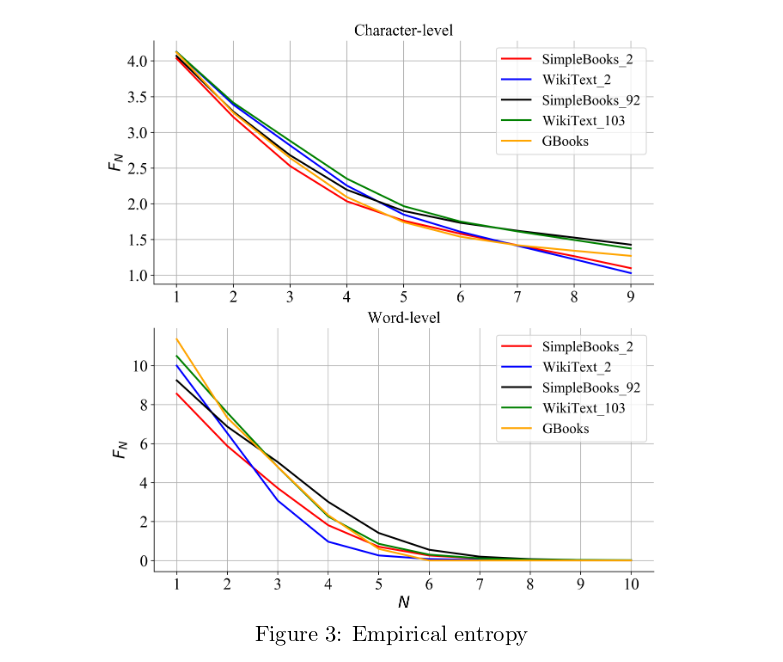## Neural networks versus N-grams language models

The values in the previous section are the intrinsic F-values calculated using the formulas proposed by Shannon. In this section, we will aim to compare the performance of word-level n-gram LMs and neural LMs on the WikiText and SimpleBooks datasets. See Table 6:

We will use KenLM  for N-gram LM. For neural LM, we use the published SOTA for WikiText and Transformer-XL [10:1] for both SimpleBooks-2 and SimpleBooks-92. This will be done by crossing entropy on the test set for both datasets.

Note that while the SOTA entropies of neural LMs are still far from the empirical entropy of English text, they perform much better than N-gram language models. The performance of N-gram language models do not improve much as N goes above 4, whereas the performance of neural language models continue improving over time. In less than two years, the SOTA perplexity on WikiText-103 for neural language models went from 40.8 to 16.4:

# The future of language modeling and language modeling evaluations

As language models are increasingly being used for the purposes of transfer learning to other NLP tasks, the intrinsic evaluation of a language model is less important than its performance on downstream tasks. Some of the downstream tasks that have been proven to benefit significantly from pre-trained language models include analyzing sentiment, recognizing textual entailment, and detecting paraphrasing. There have been several benchmarks created to evaluate models on a set of downstream include GLUE [1:1], SuperGLUE , and decaNLP .

Papers rarely publish the relationship between the cross entropy loss of their language models and how well they perform on downstream tasks, and there has not been any research done on their correlation. In the paper “XLNet: Generalized Autoregressive Pretraining for Language Understanding", the authors claim that improved performance on the language model does not always lead to improvement on the downstream tasks.

It was observed that the model still underfits the data at the end of training but continuing training did not help downstream tasks, which indicates that given the optimization algorithm, the model does not have enough capacity to fully leverage the data scale." .

But perplexity is still a useful indicator. The paper RoBERTa: A Robustly Optimized BERT Pretraining Approach shows that better “perplexity for the masked language modeling objective" leads to better “end-task accuracy" for the task of sentiment analysis and multi-genre natural language inference .

However, RoBERTa, similar to the rest of top five models currently on the leaderboard of the most popular benchmark GLUE, was pre-trained on the traditional task of language modeling. Instead, it was on the cloze task: predicting a symbol based not only on the previous symbols, but also on both left and right context. Perplexity was never defined for this task, but one can assume that having both left and right context should make it easier to make a prediction. For example, predicting the blank in “I want to __" is very hard, but predicting the blank in “I want to __ a glass of water" should be much easier. It would be interesting to study the relationship between the perplexity for the cloze task and the perplexity for the traditional language modeling task.

Although there are alternative methods to evaluate the performance of a language model, it is unlikely that perplexity would ever go away. It is a simple, versatile, and powerful metric that can be used to evaluate not only language modeling, but also for any generative task that uses cross entropy loss such as machine translation, speech recognition, open-domain dialogue.

I'd like to thank Oleksii Kuchaiev, Oleksii Hrinchuk, Boris Ginsburg, Graham Neubig, Grace Lin, Leily Rezvani, Hugh Zhang, and Andrey Kurenkov for helping me with the article.

Chip Huyen is a writer and computer scientist from Vietnam and based in Silicon Valley. She graduated with BS and MS in Computer Science from Stanford University, where she created and taught the course "TensorFlow for Deep Learning Research." She is currently with the Artificial Intelligence Applications team at NVIDIA, which is helping build new tools for companies to bring the latest Deep Learning research into production in an easier manner. Follow her on Twitter for more of her writing.

Feature image is from xkcd, and is used here as per the license.

If you enjoyed this piece and want more, subscribe to the Gradient and follow us on Twitter!

1. Alex Wang, Amapreet Singh, Julian Michael, Felix Hill, Omer Levy, and Samuel R Bowman. Glue: A multi-task benchmark and analysis platform for natural language understanding. arXiv preprint arXiv:1804.07461, 2018. ↩︎ ↩︎

2. Claude Elwood Shannon. A mathematical theory of communication. Bell system technical journal, 27(3):379–423, 1948. ↩︎

3. Claude E Shannon. Prediction and entropy of printed english. Bell system technical journal, 30(1):50–64, 1951. ↩︎ ↩︎ ↩︎

4. Equation [eq1] is from Shannon’s paper ↩︎

5. Marc Brysbaert, Michaël Stevens, Pawe l Mandera, and Emmanuel Keuleers.How many words do we know? practical estimates of vocabulary size dependent on word definition, the degree of language input and the participant’s age. Frontiers in psychology, 7:1116, 2016. ↩︎

6. William J Teahan and John G Cleary. The entropy of english using ppm-based models. In dcc, page 53. IEEE, 1996. ↩︎

7. John Cleary and Ian Witten. Data compression using adaptive coding and partial string matching. IEEE transactions on Communications, 32(4):396–402, 1984. ↩︎

8. W. J. Teahan and J. G. Cleary, "The entropy of English using PPM-based models," Proceedings of Data Compression Conference - DCC '96, Snowbird, UT, USA, 1996, pp. 53-62. doi: 10.1109/DCC.1996.488310 ↩︎

9. Matt Mahoney. Enwik8, 2006. ↩︎

10. Zihang Dai, Zhilin Yang, Yiming Yang, William W Cohen, Jaime Carbonell, Quoc V Le, and Ruslan Salakhutdinov. Transformer-xl: Attentive language models beyond a fixed-length context. arXiv preprint arXiv:1901.02860, 2019. ↩︎ ↩︎

11. Alex Graves. Generating sequences with recurrent neural networks. arXiv preprint arXiv:1308.0850, 2013. ↩︎

12. Stephen Merity, Caiming Xiong, James Bradbury, and Richard Socher. Pointer sentinel mixture models. arXiv preprint arXiv:1609.07843, 2016. ↩︎

13. Ben Krause, Emmanuel Kahembwe, Iain Murray, and Steve Renals. Dynamic evaluation of transformer language models. arXiv preprint arXiv:1904.08378, 2019. ↩︎

14. Kenneth Heafield. Kenlm: Faster and smaller language model queries. In Proceedings of the sixth workshop on statistical machine translation, pages 187–197. Association for Computational Linguistics, 2011. ↩︎

15. Alex Wang, Yada Pruksachatkun, Nikita Nangia, Amanpreet Singh, Julian Michael, Felix Hill, Omer Levy, and Samuel R Bowman. Superglue: A stick- ier benchmark for general-purpose language understanding systems. arXiv preprint arXiv:1905.00537, 2019. ↩︎

16. Bryan McCann, Nitish Shirish Keskar, Caiming Xiong, and Richard Socher. The natural language decathlon: Multitask learning as question answering. arXiv preprint arXiv:1806.08730, 2018. ↩︎

17. Zhilin Yang, Zihang Dai, Yiming Yang, Jaime Carbonell, Ruslan Salakhutdinov, and Quoc V Le. Xlnet: Generalized autoregressive pretraining for language understanding. arXiv preprint arXiv:1906.08237, 2019. ↩︎

18. Yinhan Liu, Myle Ott, Naman Goyal, Jingfei Du, Mandar Joshi, Danqi Chen, Omer Levy, Mike Lewis, Luke Zettlemoyer, and Veselin Stoyanov. Roberta: A robustly optimized bert pretraining approach. arXiv preprint arXiv:1907.11692, 2019 ↩︎

### Chip Huyen

Chip Huyen builds tools to help people productize machine learning. Find her on Twitter @chipro